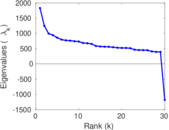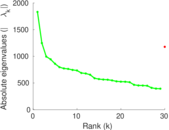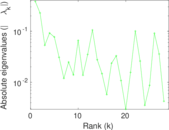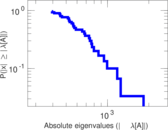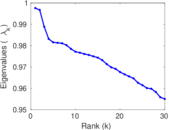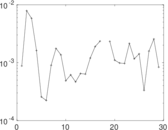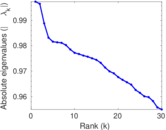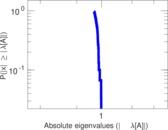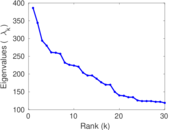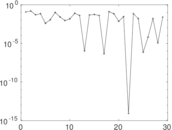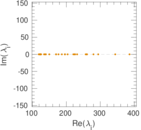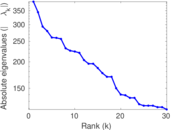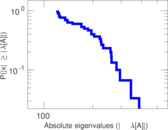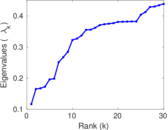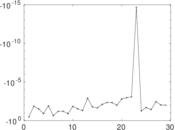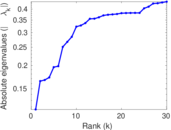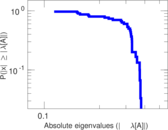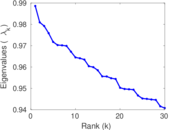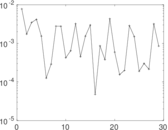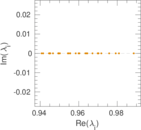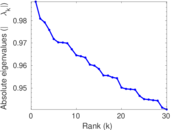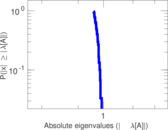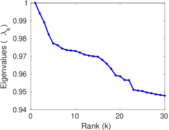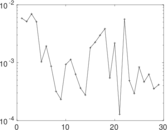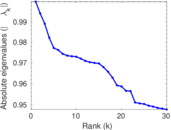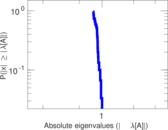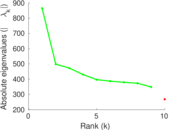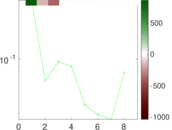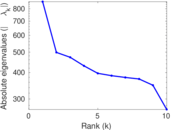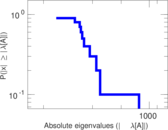This network consists of the wikilinks of the Wikipedia in the Chechen language (ce). Nodes are Wikipedia articles, and directed edges are wikilinks, i.e., hyperlinks within one wiki. In the wiki source, these are indicated with [[double brackets]]. Only pages in the article namespace are included.

 Code `Wce` Internal name `wikipedia_link_ce` Name Wikipedia links (ce) Data source http://dumps.wikimedia.org/ AvailabilityDataset is available for download Consistency checkDataset passed all tests Category Hyperlink network Node meaning Article Edge meaning Wikilink Network format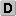Unipartite, directed Edge typeUnweighted, no multiple edges ReciprocalContains reciprocal edges Directed cyclesContains directed cycles Loops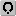Contains loops

## Statistics

 Size n = 172,283 Volume m = 7,301,226 Loop count l = 117 Wedge count s = 110,165,364,632 Claw count z = 5,002,218,494,832,529 Cross count x = 1.761 51 × 1020 Triangle count t = 347,708,660 Square count q = 614,683,977,324 Maximum degree dmax = 152,932 Maximum outdegree d+max = 1,823 Maximum indegree d−max = 152,932 Average degree d = 84.758 5 Fill p = 0.000 245 986 Size of LCC N = 172,252 Size of LSCC Ns = 76,967 Relative size of LSCC Nrs = 0.446 748 Diameter δ = 10 50-Percentile effective diameter δ0.5 = 1.606 24 90-Percentile effective diameter δ0.9 = 2.476 69 Median distance δM = 2 Mean distance δm = 2.193 71 Balanced inequality ratio P = 0.152 097 Outdegree balanced inequality ratio P+ = 0.222 968 Indegree balanced inequality ratio P− = 0.153 826 Power law exponent γ = 1.343 85 Degree assortativity ρ = −0.202 834 Degree assortativity p-value pρ = 0.000 00 In/outdegree correlation ρ± = +0.823 596 Clustering coefficient c = 0.009 468 73 Directed clustering coefficient c± = 0.947 354 Operator 2-norm ν = 1,210.55 Cyclic eigenvalue π = 909.997 Algebraic connectivity a = 0.116 378 Reciprocity y = 0.689 815 Non-bipartivity bA = 0.357 110 Normalized non-bipartivity bN = 0.070 789 6 Algebraic non-bipartivity χ = 0.116 451 Spectral bipartite frustration bK = 0.000 524 222 Controllability C = 129,905 Relative controllability Cr = 0.754 021

## Plots

### Degree distribution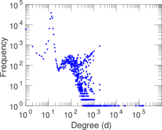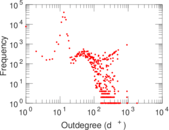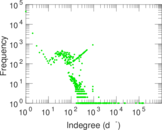### Cumulative degree distribution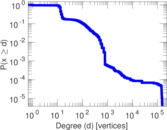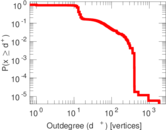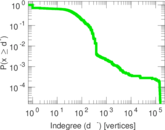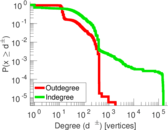### Lorenz curve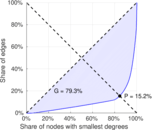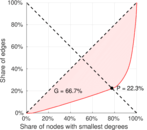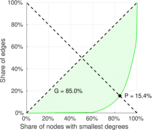### Spectral graph drawing based on the Laplacian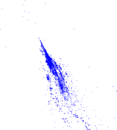### Degree assortativity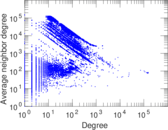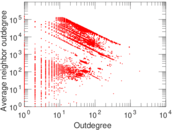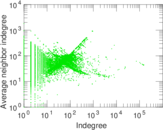### Hop distribution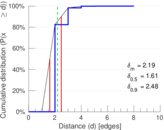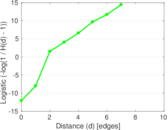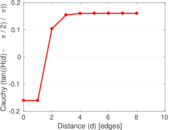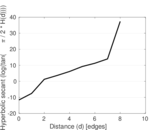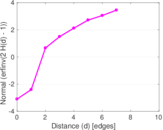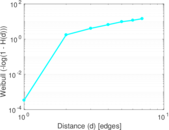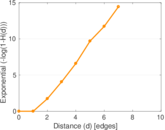### In/outdegree scatter plot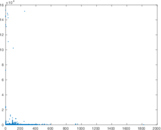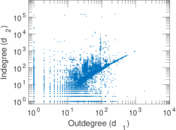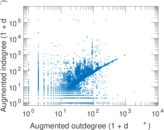### SynGraphy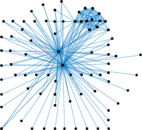### Matrix decompositions plots